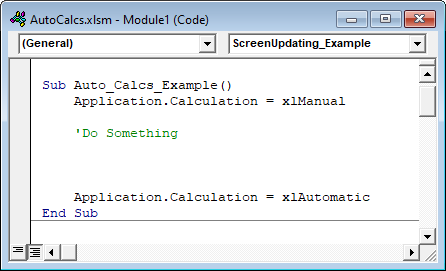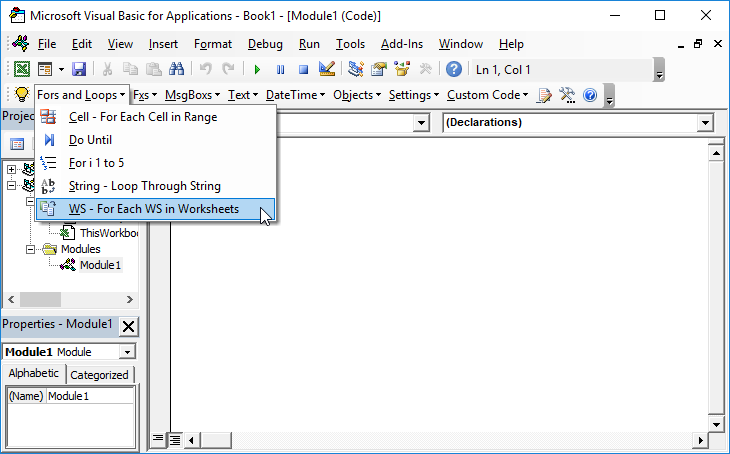# VBA – Turn Automatic Calculations Off (or On)

Whenever you update a cell value, Excel goes through a process to recalculate the workbook. When working directly within Excel you want this to happen 99.9% of the time (the exception being if you are working with an extremely large workbook). However, this can really slow down your VBA code. It’s a good practice to set your calculations to manual at the beginning of macros and restore calculations at the end of macros. If you need to recalculate the workbook you can manually tell Excel to calculate.

## Turn Off Automatic Calculations

You can turn off automatic calculation with a macro by setting it to xlmanual. Use the following piece of VBA code:

``Application.Calculation = xlManual``

## Turn Automatic Calculations Back On

To turn back on automatic calculation with the setting xlAutomatic:

``Application.Calculation = xlAutomatic``

I recommend disabling Automatic calculations at the very beginning of your procedure and re-enabling Automatic Calculations at the end. It will look like this:

## Disable Automatic Calculations Macro Example

``````Sub Auto_Calcs_Example()
Application.Calculation = xlManual

'Do something

Application.Calculation = xlAutomatic
End Sub``````## Manual Calculation

When Automatic calculations are disabled, you can use the Calculate command to force Excel to recalculate:

``Calculate``

You can also tell Excel to recalculate only an individual worksheet:

``Worksheets("sheet1").Calculate``

You can also tell VBA to recalculate just a range (click to read our article about VBA calculation methods)

Here is how this might look inside a macro:

``````Sub Auto_Calcs_Example_Manual_Calc()
Application.Calculation = xlManual

'Do Something

'Recalc
Calculate

'Do More Things

Application.Calculation = xlAutomatic
End Sub``````

### VBA Settings – Speed Up Code

If your goal is to speed up your code, you should also consider adjusting these other settings:

Disabling Screenupdating can make a huge difference in speed:

``Application.ScreenUpdating = False``

Turning off the Status Bar will also make a small difference:

``Application.DisplayStatusBar = False``

If your workbook contains events you should also disable events at the start of your procedures (to speed up code and to prevent endless loops!):

``Application.EnableEvents = False``

Last, your VBA code can be slowed down when Excel tries to re-calculate page breaks (Note: not all procedures will be impacted).  To turn off DisplayPageBreaks use this line of code:

``ActiveSheet.DisplayPageBreaks = False``

Stop searching for VBA code online. Learn more about AutoMacro – A VBA Code Builder that allows beginners to code procedures from scratch with minimal coding knowledge and with many time-saving features for all users!